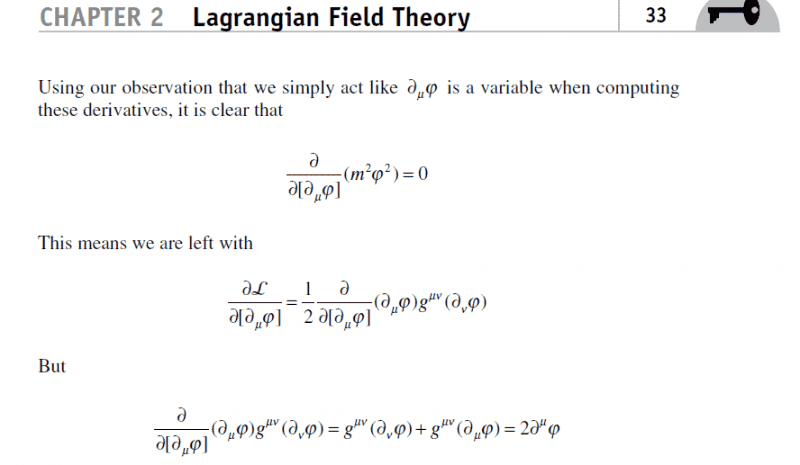# Equation Derivation in QFT book by D.MacMahonhow?

• Abolaban
It is mainly a matter of understanding index notation, if you do that, you should be able to follow - of course assuming derivation is corrected to:$$\frac{\partial \mathcal L}{\partial(\partial_\rho\varphi)} = \frac{\partial}{\partial(\partial_\rho\varphi)} g^{\mu\nu}(\partial_\mu\varphi)(\partial_\nu\varphi) = g^{\mu\nu}[ \delta_\mu^\rho \partial_\nu\varphi + \delta_\nu^\rho\partial_\mu\varphi] = 2\f #### Abolaban Hello, I have encountered an equation in page 33 in the book of D.MacMahon titled QFT demystefied. It is the third equation from the top...how did the sum appear as a middle step of the equation?best regards. Abolaban Ok, so this is very bad notational form. He is using ##\mu## as the index of the ##\partial_\mu## at the same time as he is using it as a summation index in the Lagrangian. The middle step is simply wrong - you can never (ever ever) have an expression where you sum two terms with different free indices! What should have been done is to pick a different summation index for the Lagrangian (or why not differentiate with respect to ##\partial_\rho\varphi##?). You can then apply the rules for a derivative of a product and note that ##\partial(\partial_\mu \varphi)/\partial(\partial_\rho \varphi) = \delta_\mu^\rho##. Edit: To be clear, the end result is correct, the middle step is not. •vanhees71 Thank you "Orodruin" for your answer... where can one correctly learn about the usuage of such notations? where can one correctly learn about the usuage of such notations? I don't know any book that would go into showing calculations as Demystified... In most of cases you have to be very careful yourself of the writer's steps and what he is actually doing... eg if you did the same calculation yourself, you wouldn't use same-indices with the derivatives. Edit: To be clear, the end result is correct, the middle step is not. :D Correct by luck... If you are familiar with tensor notation, there really is not much more to it other than seeing ##\partial_\mu \varphi## for different values of ##\mu## as independent arguments of the Lagrangian. It should then be rather obvious that, for example, ##\partial(\partial_1\varphi)/\partial(\partial_1\varphi) = 1## and that ##\partial(\partial_1\varphi)/\partial(\partial_2\varphi) = 0##, etc. :D Correct by luck... The best (i.e., worst) way of being correct. Although I think it is rather a case of "IknowwhatIshouldgetsoIwillbesloppyinthemiddlesteps"-syndrome. thanks "Oroduin" for your comment... I have the following books, however, it is hard to recognize which could match the QFT tensor analysis...what do you recommend among them? -Daniel Fleisch: A Student's Guide to Vectors and Tensors -Derek F.Lawden: An Introduction to Tensor Calcul -J.A.Schouten:Tensor Analysis for Physicists -Mikhail Itskov: Tensor Algebra and Tensor Analysi -Nadir Jeevanjee: An Introduction to Tensors -Nazrul Islam: Tensors and their applications Abolaban It is mainly a matter of understanding index notation, if you do that, you should be able to follow - of course assuming derivation is corrected to:$$
\frac{\partial \mathcal L}{\partial(\partial_\rho\varphi)} = \frac{\partial}{\partial(\partial_\rho\varphi)} g^{\mu\nu}(\partial_\mu\varphi)(\partial_\nu\varphi)
= g^{\mu\nu}[ \delta_\mu^\rho \partial_\nu\varphi + \delta_\nu^\rho\partial_\mu\varphi] = 2\partial^\rho\varphi
$$•vanhees71 "Orodruin" thanks for your further clarification... I went through "Tensor analysis" in Math Meth for Physicists by Arfken and Weber pages 133 and beyond but I could not recognize how did you split that term into summation!? "Orodruin" thanks for your further clarification... I went through "Tensor analysis" in Math Meth for Physicists by Arfken and Weber pages 133 and beyond but I could not recognize how did you split that term into summation!? Do you understand the following result for ordinary derivatives of functions of one variable?$$
\frac{d}{dx}f(x) g(x) = f'(x) g(x) + f(x) g'(x)


I went through "Tensor analysis" in Math Meth for Physicists by Arfken and Weber pages 133 and beyond but I could not recognize how did you split that term into summation!?

there will be more that look similar to this, but this is straightforward to see... equation 2.130b he uses the same "summation" thing, generally called the product rule...

yeah...you use this from vector analysis...dealing with tensors carry special flavours...so the tast might sometimes mix in one's tongue.

--equation 2.130b seems to carry different flavour...namely by Christoffel

--equation 2.130b seems to carry different flavour...namely by Christoffel

flavour?

Well what he did was write $\textbf{V} = V^i e_i$
and take the derivative $\frac{d}{dq^j} \textbf{V} =\frac{d}{dq^j} (V^i e_i) =\frac{dV^i}{dq^j} e_i + V^i \frac{de_i}{dq^j}$

Especially at this point, it is just vector-analysis...

yeah...you are correct...it is the same...however using sub and super scripts while dealing with McMahon's notation is sometimes confusing...

He is using for both subscripts because he is taking the derivative with respect to the $\partial_\mu \phi$ (see the subsript).
The kinetic term can as well be written by using the metric:
$(\partial_{\mu} \phi) g^{\mu \nu} (\partial_\nu \phi) = (\partial_\mu \phi) (\partial^\mu \phi ) =(\partial^\mu \phi ) (\partial_\mu \phi)$

But using just the metric he can easily use the:
$\frac{\partial (\partial_{\nu} \phi) }{\partial (\partial_\mu \phi)} = \delta_{\nu}^\mu$.

$\frac{\partial (\partial^{\nu} \phi) }{\partial (\partial_\mu \phi)} = g^{\nu \rho} \delta_{\rho}^\mu$.